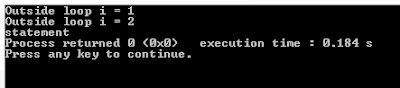Break statement in C is a loop control statement. This statement terminates the loop. As soon as the break statement found in the loop in C program, it ends loop iteration. And the control goes to the first statement after the loop.

The break statement is used in looping statement and in switch statement in C.

### Syntax

for (initialization; test_condition; increment)
{
statement;
if (test_condition)
{
statement1;
break;
}
statement 2;
}

If test_condition written within the loop is true, then statement1 and break will execute. The break statement in C terminates the loop (loop execution will stop). Program control will move to statement2.

For instance,
#include <stdio.h>
int main()
{
int i=0; // Initialize variable i
for (i=1;i<5;i++)

{
if(i==3)
{
printf ("statement");
break;
}
printf ("Outside loop i = %d\n",i);
}
return 0;
}

### Output## Use of Break Statement in C

1. When the break statement is encountered in the loop, then the loop is terminated. And the program control will execute the statements after the loop.

2. You can use the break statement in the switch statement in C.
3. If you use a break statement in a nested loop, then the break statement stops the execution of the innermost loop. And execute the statements after the loop.

## How Break Statement in C Works?

As soon as the break statement occurred in the program. Then, it stops the execution of the loop. And execute the statements written after the loop. For instance,

## Example of Break Statement in C with Switch Statement

Click here to learn Use of break statement in switch statement in C.

#include <stdio.h>
int main()
{
int a =10, b=5; //Initialization of Variables
switch (a>b)
{
case 1:
printf ("a is greater than b");
break;
case 0:
printf ("b is greater than a");
break;
default:
printf ("Print value of a and b %d%d\n", a,b);
}
return 0;
}

### Output

a is greater than b

## Break Statement in C with While loop

Learn with the help of the C program to use the break statement in the while loop.
#include <stdio.h>
int main()
{
int j=5; //initialization of variable
while (1)
{
printf ("%d", j);
j--;
if (j==0)
break;
}
printf ("Out of the loop");
return 0;
}

5
4
3
2
1
Out of the loop

## Break Statement in C with a do-while loop

Learn with the program how to break statement used in the do while loop in C.
#include <stdio.h>
int main ()
{
int a=5,j,choice; //Initialization of variables
do
{
j=1;
while(j<=10)
{
printf("%d X %d = %d\n",a,j,a*j);
j++;
}
printf("Enter positive value %d\n",a+1);
scanf("%d",&choice);
if(choice == 0)
{
break;
}
a++;
}while(1);
printf ("Outside the loop");
return 0;
}

### Output

5 × 1 = 5
5 × 2 = 10
5 × 3 = 15
5 × 4 = 20
5 × 5 = 25
5 × 6 = 30
5 × 7 = 35
5 × 8 = 40
5 × 9 = 45
5 × 10 = 50
Enter positive value 6

## Break Statement in C with Nested Loop

#include <stdio.h>
int main()
{
int i=1, j=1; //Initialization of variable
for (i=1;i<=4;i++)
{
for (j=1;j<=4;j++)
{
printf ("%d %d\n", i,j);
}
if(i==3 && j==3)
{
break; //End j loop
}
printf ("End of i loop\n");
break; //End i loop
}
printf ("Last statement");
return 0;
}

1 1
1 2
1 3
1 4
End of i loop
Last statement

## What is the Difference Between Break and Continue Statement in C?

 S.No. Break Statement Continue Statement 1 The break statement in C is used in both loop (for, while and do-while) and in switch statement in C. This statement is used only in Loop statements like For, while and do-while loop. 2 As soon as the break statement has occurred in the loop, it stops the execution of the loop abruptly. While the Continue Statement does not terminate the program. All iterations of the loop are executed even if the continue statement is encountered.
Learn with Video What is Break Statement in C? (Deep Discussion)Reviewed by Glory on 10:03 Rating: 5